### Journal of Current Scientific Research

Current Issue Volume No: 1 Issue No: 2

ISSN: 2766-8681
share this page

Research Article Open Access
• Available online freely Peer Reviewed
• Provisional

### Nature Inspired Bargain Optimization Algorithm for Effective Interpretation of Geoelectrical Data

Stanley Raj 1   J.P. Angelena 1   D. Senthil Kumar 2   S. Akshaya 3   R. Dhamodharan 4   M. Hariharan 1

1Department of Physics, Loyola College, Chennai, Tamil Nadu-India

2Department of Physics, School of Science and Humanities, Vel Tech Rangarajan Dr. Sagunthala R&D Institute of Science and Technology, Avadi , Chennai 600 062, Tamil Nadu, India

3Department of Physics, SDNB Vaishnav College, Chrompet, Chennai,- 600 044, India

4Centre for Geotechnology, Manonmaniam Sundaranar University, Tamil Nadu, Tirunelveli

## Abstract

Geoelectrical resistivity data collected from the ground contain lot of noises and errors. It requires efficient algorithm to reduce the errors to make an actual inversion models. Though different algorithm can be applied, nature inspired algorithm is more potential in inverting geoelectrical data in an elegant and comprehensive way. Bargain Optimization (BO) algorithm is framed on the concept of bargaining things to purchase for needs. In general, effective bargaining results in more profit and leads to loss when it fails. In this research work, Bargain Optimization algorithm is applied to invert geoelectrical data and the effective bargaining will take time to process and to obtain the required model. The input data is AB/2, apparent resistivity data and the inverted model through BO algorithm is successfully matched with the available litholog section of the study area. The output graphs have profit/loss bar graph, which reveals the status of bargaining during a particular number of epochs.

##### Author Contributions
Received 02 Apr 2021; Accepted 22 May 2021; Published 04 Jun 2021;

Copyright ©  2021 A. Stanley Raj, et al.

LicenseThis is an open-access article distributed under the terms of the Creative Commons Attribution License, which permits unrestricted use, distribution, and reproduction in any medium, provided the original author and source are credited.

Competing interests

The authors have declared that no competing interests exist.

Citation:

Stanley Raj, J.P. Angelena, D. Senthil Kumar, S. Akshaya, R. Dhamodharan et al. (2021) Nature Inspired Bargain Optimization Algorithm for Effective Interpretation of Geoelectrical Data. Journal of Current Scientific Research - 1(2):24-34.

Download as RIS, BibTeX, Text (Include abstract )

## Introduction

Groundwater plays vital role in our ecosystem as it replenishes lakes, rivers wetlands etc., and used for principal source of drinking water and it is also utilized for industrial and agricultural purposes. The significant escalation of human activities and various reasons such as climate change, the global groundwater resources are under large stress. The stable advancement of various geophysical techniques with the substantial usage of different physical properties for the application of ground water exploration are electrical resistivity, magnetic susceptibility, elasticity, density and radioactivity 10, 20. Among the various kinds of geophysical prospecting techniques, the geoelectrical resistivity method has become a significant tool for groundwater exploration 21.

The Electrical resistivity method has usually been employed in determining the model parameters of the subsurface of our Earth 1. Globally, Direct current resistivity methods of geoelectrical prospecting method are greatly employed for assessment of various aquifer parameters such as thickness and resistivity 9, 15, 17. The interpretation of geoelectrical resistivity data is essential to recognize the idea of certainty in the subsurface system of the Earth and there is in need of an effective tool to guesstimate and evaluate the parameters which are appropriately related to the subsurface system. The optimization of geoelectrical resistivity inverse problems needs a suitable association between mathematical models and the physical model parameters. The evaluation of model parameters of the subsurface layer of the Earth has been effectively estimated with the incorporation of a powerful tool 13.

The process of optimization is one of the best techniques to evaluate the results. Basically, optimization involves in minimizing the errors between the both anticipated and observed results within the peculiar constraints. Several researchers applied neural networks coupled with other optimization algorithm to produce favorable results 5, 6, 7. The Inputs defined, are of numerous variables that the function is framed into certain conditions to yield appreciable results. There are several optimization techniques that are nature inspired algorithms. Artificial Neural networks (ANN)is one of the biomimicking algorithm that estimates the result on the basis of training progress and It shows its immense mapping proficiencies effectively between the input and output patterns. Since ANN learns through better framed examples, the training dataset was established synthetically and have been tested. The evident layer model delivers the information about the thickness and true resistivity of the subsurface layer 18.

Artificial neural networks have independent-learning competence and are of noise-immune and founds applications in numerous fields 11, 14. Many researchers 3, 11, 15, 16 utilized ANN as an optimization tool for solving various geophysical problems. To an extent, various geophysical prospecting methods can be improved to congregate the number of solutions for inverse problems. To understand lithological constraint Bosch and 2 used gravity and magnetic prospecting methods which yields better results. Seismic prospecting method have been employed to estimate geophysical characteristics by 4, 8, 12 have done inversion to interpret geophysical data.

## Methodology

Bargain optimization algorithm has been applied here for inverting geoelectrical data 19

## Initialization

A) Feeding input data

B) Set up minimum error percent

C) Set up time limit

In this process of initialization any nonlinear data can be feed as an input. Here in this article geoelectrical resistivity data obtained from different field data has been applied to evaluate the algorithm. As geology varies from region to region, electrical resistivity data obtained from the field is completely non-linear. It depends on many parameters, viz., porosity, humidity of the soil, atmospheric variations, etc. If the subsurface geology is very complex the resistivity variations can rapidly vary over short distances. Table 1 gives the resistivity values of common rocks, soil materials and chemicals (Keller and Frischknecht 1966, Daniels and Alberty 1966).

Table 1. Performance of different types of algorithm in comparison with BO algorithm
 S. No Algorithm MSE PSNR R Value RMSE NRMSE MAPE COMPUTATIONAL TIME 1. Feedforward 65.03 29.9 0.98 8.064 0.0698 11.22 125.25 2. Radial basis network 7.8 31.9 0.98 2.79 2.41 4.9 6.32 3. Exact Radial Basis network 8.1 31.9 0.98 2.8 2.46 5.19 13.2 4. Generalised regression neural networks 0.10 57.9 0.97 0.32 0.21 0.48 3.4 5. Probabilistic neural networks 6.78 9.8 0.94 81.9 0.70 98.1 2.99 6. Bargain Optimization 5.4 4.0 0.99 2.3 0.02 4.9 2.3

Thus, setting up the minimum error percent and time required for ‘bargaining’ is very important. Bargaining concept is very similar to training in neural networks but exclusively novel in the concept of utilizing weights and profit/loss conception.

## Finding Tolerance Level

In this step algorithm find the difference between each data and the mean difference is fixed as the tolerance level. For a sample data size of n, mean absolute deviation can be calculated as the tolerance level,

i Is the mean of distribution.

This will be helpful in bringing the optimal solution for the problem, because the convergence rate and weight based learning is within this tolerance level. This step is very important to prepare the data for bargaining process

## Process of Bargaining

This process starts with all the prerequisites of the algorithm and the systematic weight based learning starts here at this step. Systematic weight learning method is the one which adds weights to the data to form a synthetic data for learning purpose. Thus in each iteration process of bargaining follows a ‘weight reduction technique’ i.e., If the weights added up is very close or within the tolerance level then the data with added weights will not appear in the next iteration. This saves the time in learning process. Continuous bargaining results in effective time bound learning methodology with profit/loss. Moreover, the technique of bargaining may result failure in some attempts of bargaining and it has been recorded as bargain chart which clearly mentions the bargain failure at specific iteration.

## Relative Variation (Statistical analysis)

Finding the mean for sample data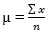Where ‘x’ is the data and ‘n’ represents the number of data points

Standard deviation for the data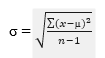Coefficient of variation (CV) can be calculated as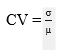This step checks the relative variation between the synthetic data and the field data taken for study. The uncertainties involved in the data process can be analyzed using this relative variation.

## Profit/ Loss

The algorithm checks with the permissible error percent and conditions the loop to break or to proceed. Profit can be obtained if the data fits in the tolerance value but the accuracy and precision is based on the error percent. Thus the algorithm may continue its iteration though small amount of profit is obtained. The algorithm continuously iterating until it attains the maximum profit (the desirable one)

## Performance Evaluation

L2 – norm is the performance evaluation based on least square estimates. It is basically minimizing the sum of square of the differences (E) between the target (Yi) and estimated values (f(xi)

## Feed forward Technique

A feedforward neural network is a sorting method motivated by biology. It is made up of a (probably massive) number of basic neuron-like computing modules that are arranged in layers. Per unit in a layer is linked to a unit in the preceding stage. These relations are never exactly the same: each one might be of varying intensity or weight. The weights on these links encode a channel's information. The modules in a neural network are frequently referred to as nodes.

Data joins the system at the inputs and travels through the network, layer by layer, until it reaches the outcomes. There really is no feedback among layers throughout normal activity, that is, when it serves as a classification model. This is why they're referred to as feedforward neural networks.

## Radial Basis Function Network

At its most basic form, an RBF network is a three-layer feedforward neural network. The first layer contributes to the network's inputs, the second is a concealed layer made up of a series of RBF non-linear activation modules, and the third contributes to the network's overall outcome.   RBFN trigger functions are typically configured as Gaussian functions. The general form of RBF is

Where, K is a positive non linear symmetric radial function; X is the input pattern and µ is the centre of the function.

## Generalised Regression Neural Network

One among the most common neural networks is GRNN, which is a form of regulated FFNN. The GRNN architecture is made up of four layers: The first layer serves as the input layer and is fully wired to the second layer. The second layer is the first one that is covered (also called the pattern layer). The second secret layer (Summation layer) has two nodes in the third layer. The output layer is the fourth layer. It takes the two hidden layer outputs and splits them to get an approximation for y. (or to provide the prediction result).

Let f(x, y) represent the continuous probability density function of a vector random variable, X, and a scalar random variable, Y. Let x represent a specific calculated value of the random X. The regression of Y given x (also known as the conditional variance of Y given x) is calculated as follows

## Probablistic Neural Network

Pnns are frequently more reliable than ffnns, and training pnns is often easier than training ffnns. The most significant benefits of pnns are the reality that the outcome is probabilistic, making analysis of the performance simple, and the training frequency. PNN's basic composition consists of four layers. The input layer is the initial layer The pattern layer is. The secondary level.  The summation layer is the next layer.  The output layer is the final layer (also called decision layer). The use of PNN is particularly useful because of its capacity to correlate to the fundamental function of the dataset with only a small number of training samples present. The output of the ith pattern neuron in the kth group is computed using a Gaussian kernel of the form

Where, i is the pattern number.

P denotes the dimension of pattern vector x.

σ is the smoothing parameter of Gaussian kernel.

Xaii is the centre of kernel.

## Results and Discussion

Intelligent data analysis can interpret geophysical data with accurate and plausible results. Though the geophysical parameter involves lot of noises and errors, intelligent data analysis can filter and manage the data to provide optimized solution. Geoelectrical data is one of the such kind with noises from heterogeneous media of earth. This errors and noises will suppress the original sub surface geology of the data.

Table 1 shows the performance of different types of algorithm in comparison with BO algorithm. The table shows the performance of Feed forward, Radial basis Network, Exact Radial Basis network, Generalised Regression Neural Network, Probabilistic Neural Network in comparison with BO algorithm.

The values of MSE, PSNR, R- Value, RMSE (Root Mean Square Error), NRMSE, MAPE, Computational Time. The comparison of the performance function from different algorithm with the Bargaining Optimization algorithm is stated below.

## Mean Square Root (MSE)

In General, the mean squared error (MSE) of an optimization technique in statistics calculates the sum of the squares of the errors—that is, the average squared discrepancy between the expected and real values.

Where,

N – Number of training data

Di – Desired Output Value

Oi – ANN’s Output Value

The MSE value of Feedforward Network is much higher when compared to other algorithms. The MSE obtained from Generalized Regression Neural Network is 0.10 representing that the algorithm is much accurate than other techniques. BO algorithm is the second most accurate technique which states that it is better for prediction.

## Peak Sound to Noise Ratio

Peak signal-to-noise ratio (PSNR) is an equation for the ratio of a signal's highest potential value (power) to the power of altering noise that influences the accuracy of its representation.

According to the obtained PSNR values, Generalized Regression Neural Network has the highest value of PSNR ratio with 57.9.

## R – Value

The coefficient of correlation is denoted by the letter R. It indicates how well the expected outputs align with actual outputs, with R close to 1 indicating a good qualified network and 0.2 and 0.3 indicating a poor network.  The R values of all the algorithm are nearly equal to 1.

## Root Mean Square Error (RMSE)

The root of the mean square error value gives the RMSE values.

RMSE has never been negative, and a value of 0 (which is almost never obtained in reality) indicates a great match to the results. In general, a lower RMSE is preferable to a higher RMSEThe value of RMSE is BO algorithm is lower than all the other algorithms, which indicated that the Bargaining Optimization is more preferable to predict the values.

## Normalized root mean square error (NRMSE)

Where,

P = number of output processing elements

Di – Desired Output Value

MSE- Mean Squared error

## Mean Absolute Percentage Error (MAPE)

Since MAPE is a calculation of error, higher values are weak and lower values are great.

Where,

At – Actual Value

Ft – Forecasted Value

The MAPE value we got for BO algorithm is less than 10 percentage, which shows that the technique is very much accurate. Whereas the Feedforward and probabilistic neural network has the higher values of MAPE which corresponds to less accuracy.

## Computational Time

The amount of time needed to complete a computing task is referred to as computation time. The computational time for feedforward technique is more time taking whereas the Bargaining Optimization Algorithm is the fastest algorithm which gives the most approximate outcome.

Figure 1. Geology of the study area (13.1382° N, 79.9071° E)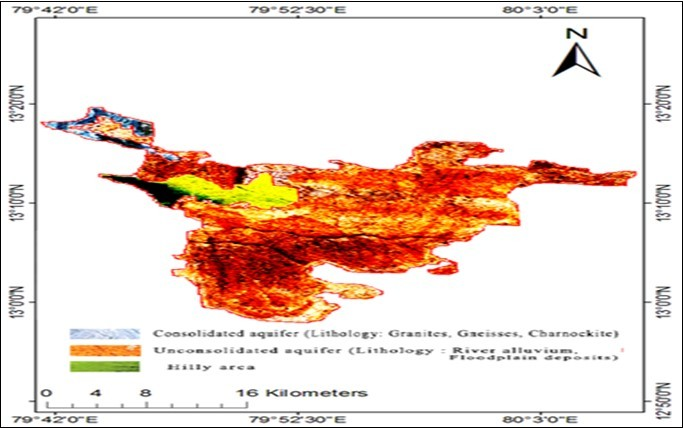Figure 2. Main Panel for inverting geoelectrical data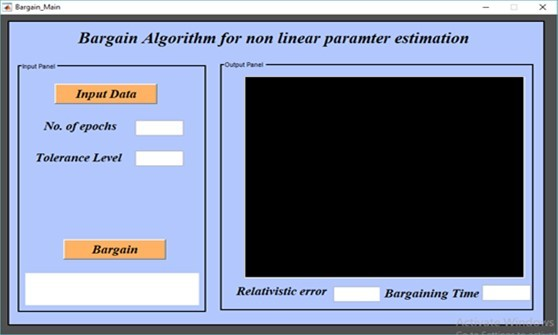Figure 3. Inversion of Geoelectrical data using BO algorithm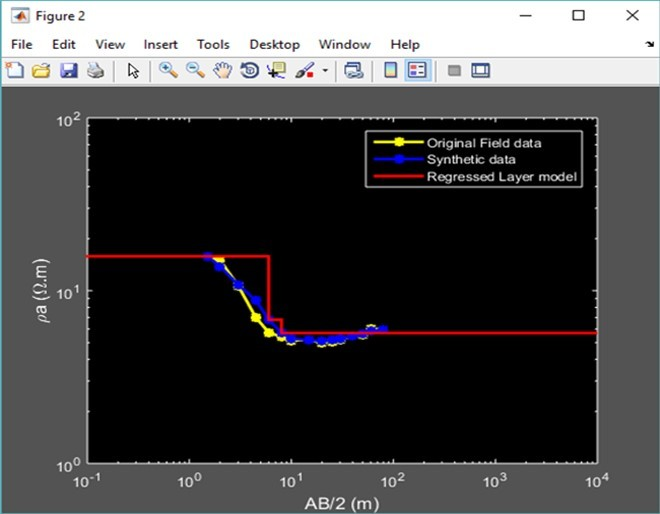Figure 4. Profit/ loss- Graphical representation of Data 1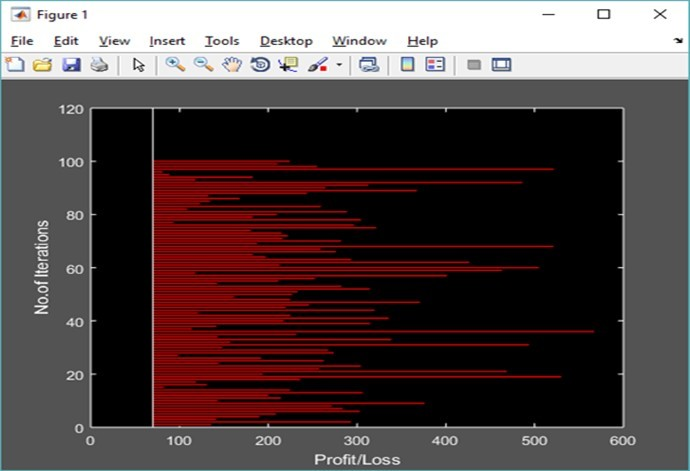Figure 5. Main panel for inverting geoelectrical data (Data 2)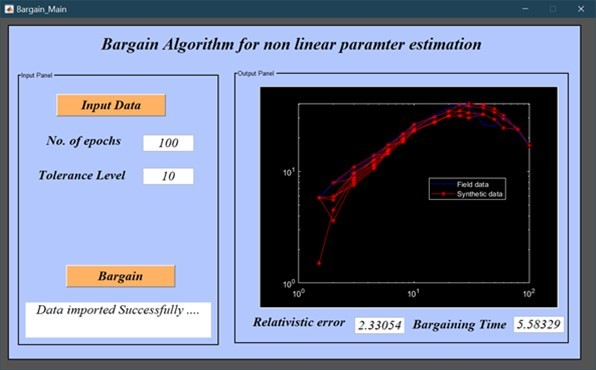Figure 6. Profit/ loss- Graphical representation of Data 2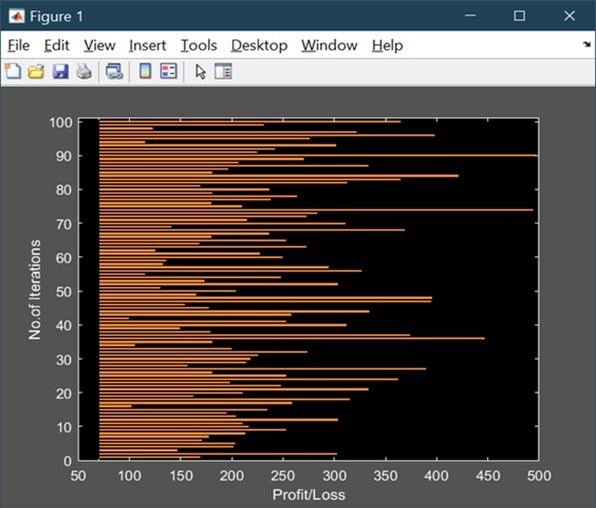Figure 7. Inverted geoelectrical model for data2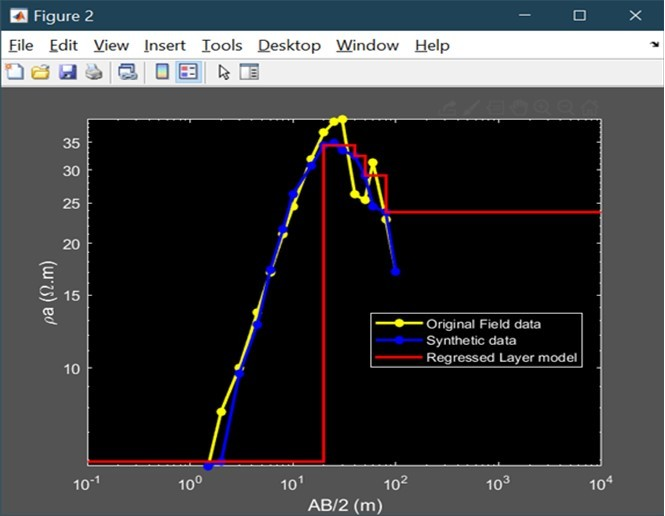Figure 8. Litholog section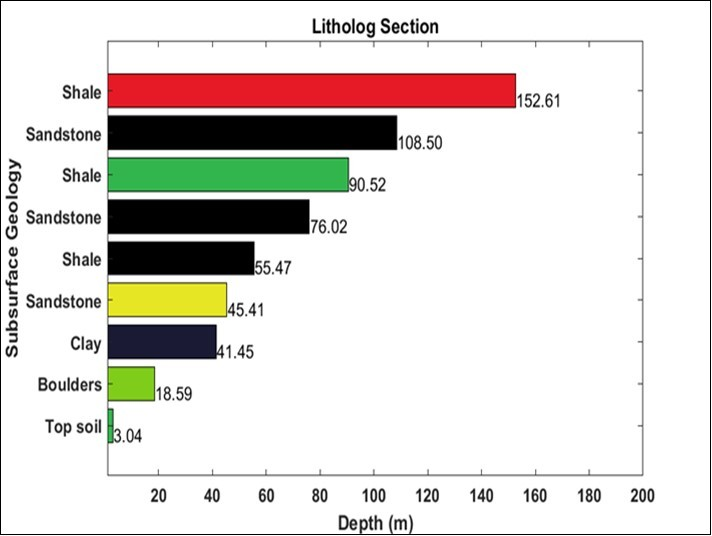Figure 1 represents the geology of the study area. Figure 2 shows the Graphical User Interface (GUI) of bargain optimization algorithm for inverting geoelectrical data. The main panel contains the push button for importing data. The user can give the number of epochs and tolerance level for training the data. After successful bargaining, the system will provide the geoelectrical model with relativistic error and bargaining time. Figure 3 shows that inversion of geoelectrical data 1. The profit/ loss diagram is shown in Figure 4. This diagram explains about the concept of bargaining .If the bargaining is successful the profit will be more during the number of iterations. If the bargaining fails, loss will be more and the bargaining time will also increase. Relativistic error will represent the difference between the original and the synthetic field data. Figure 5 represents the main panel for inverting geoelectrical data2. Figure 6 and Figure 7 represents the profit/loss diagram and the inverted geoelectrical model respectively. Figure 8 represents lithology section of the study area.